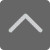## 爱智康资讯站

### 课程咨询: 4000-121-121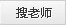# 2018-2019学年度一年级暑假作业及答案 数学学科

2019-07-30 05:58:12 　来源：本站原创

2018-2019学年度暑假到来了，武汉学而思爱智康为大家收集整理了人教版的暑假作业资源2018-2019学年度一年级暑假作业及答案 数学学科的相关内容，方便大家学习参考哟。

2019年人教版一年级数学暑假作业题。面对危险，其它羚羊像老羚羊那样凌空腾起，没有拥挤，没有争夺，秩序井然，快速飞跃。这里有成都学而思一对一小编为大家收集整理了2019年人教版一年级数学暑假作业题，希望能够对同学们有所帮助。

一、计算

30+47=

24+9=

3+46=

45-5=

12-9=70-60=

41+6=

40-8=

6+5-4=

83-30=

39+1=

65-40=

8+24=

30+10+7=

10+18=

6+14=

60-20-40=

10+59=

4+6+1=

8+11=

二、应用题

1、小红剪五角星，第一次剪了15个，第二次剪的和第一次剪的同样多。两次一共剪了多少个?

2、福娃贝贝和晶晶参加50米的赛跑。贝贝用12秒，晶晶用11秒。谁跑得快?快多少秒?

3、妈妈拿一张50元的钱，买了一本25元的字典和一本15元的百科知识，应找回多少元?

小学一年级数学暑假作业【篇二】

一、计算

3+48=

7+74=

43+9=

46+6=

65+7=26+9=

17+6+8=

23+4+9=

62-50+7=

50+27-8=

50+50=

100-50=

20+40=

50+30=

50+20-30=

90-70+60=

16-6=

4+23=

33+6=

7+5=

二、应用题

1、一年级有98个同学去旅游。第一辆车只能坐40人，第二辆车能坐55人。还有多少人不能上车?

2、一年级有56人参加游园比赛。在第一轮比赛中，有28人走了，又来了37人参加第二轮比赛。现在有多少人参加游园比赛?

3、一双球鞋的价格是72元，一双布鞋的价格比一双球鞋的价格便宜了48元。一双布鞋的价格是多少元?

小学一年级数学暑假作业【篇三】

一、计算

18+5+9=

59-8+20=

16+20-30=

6+2=

0+6=

84+6-1=

40+15-14=

5+3=

7+2=

8-5=

3+6=

8-4=

10-4=

5+5=

8+0=

9+7=

7+3=

8-6=

9-5=

3+2=

二、应用题

1、小兰今年9岁，妈妈今年36岁，妈妈和小兰相差多少岁?

2、工人叔叔修路，第二天比第一天多修14米，第一天修62米，第二天修路多少米?

3、一双球鞋21元，一双布鞋比一双球鞋便宜9元，一双布鞋多少元?买一双球鞋和一双布鞋要用多少元?

以上就是学而思爱智康为大家整理的2018-2019学年度一年级暑假作业及答案 数学学科的相关内容，如需诊断学习模块的掌握情况，获取学习方面的指导帮助，可拨打电话：4000-121-121咨询。学而思爱智康课程官方报课渠道：www.izhikang.com 。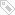标签：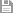保存　｜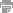打印　｜关闭